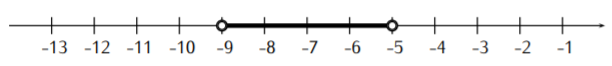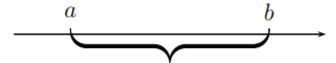# 1.4: Absolute value inequalities

$$\newcommand{\vecs}{\overset { \rightharpoonup} {\mathbf{#1}} }$$ $$\newcommand{\vecd}{\overset{-\!-\!\rightharpoonup}{\vphantom{a}\smash {#1}}}$$$$\newcommand{\id}{\mathrm{id}}$$ $$\newcommand{\Span}{\mathrm{span}}$$ $$\newcommand{\kernel}{\mathrm{null}\,}$$ $$\newcommand{\range}{\mathrm{range}\,}$$ $$\newcommand{\RealPart}{\mathrm{Re}}$$ $$\newcommand{\ImaginaryPart}{\mathrm{Im}}$$ $$\newcommand{\Argument}{\mathrm{Arg}}$$ $$\newcommand{\norm}{\| #1 \|}$$ $$\newcommand{\inner}{\langle #1, #2 \rangle}$$ $$\newcommand{\Span}{\mathrm{span}}$$ $$\newcommand{\id}{\mathrm{id}}$$ $$\newcommand{\Span}{\mathrm{span}}$$ $$\newcommand{\kernel}{\mathrm{null}\,}$$ $$\newcommand{\range}{\mathrm{range}\,}$$ $$\newcommand{\RealPart}{\mathrm{Re}}$$ $$\newcommand{\ImaginaryPart}{\mathrm{Im}}$$ $$\newcommand{\Argument}{\mathrm{Arg}}$$ $$\newcommand{\norm}{\| #1 \|}$$ $$\newcommand{\inner}{\langle #1, #2 \rangle}$$ $$\newcommand{\Span}{\mathrm{span}}$$$$\newcommand{\AA}{\unicode[.8,0]{x212B}}$$

Using the notation from the previous section, we now solve inequalities involving the absolute value. These inequalities may be solved in three steps:

• Step 1: Solve the corresponding equality. The solution of the equality divides the real number line into several subintervals.
• Step 2: Using step 1, check the inequality for a number in each of the subintervals. This check determines the intervals of the solution set.
• Step 3: Check the endpoints of the intervals.

Here are some examples for the above solution method.

## Example $$\PageIndex{1}$$

Solve for $$x$$:

1. $$|x+7|<2$$
2. $$|3x-5|\geq 11$$
3. $$|12-5x|\leq 1$$

Solution

1. We follow the three steps described above. In step 1, we solve the corresponding equality, $$|x+7|=2$$. $$x+7=2$$ & $$x+7=-2$$ $\begin{array}{l|l} x+7=2 & x+7=-2 \\ \Longrightarrow x=-5 & \Longrightarrow x=-9 \end{array} \nonumber$
The solutions $$x=-5$$ and $$x=-9$$ divide the number line into three subintervals:

Now, in step 2, we check the inequality for one number in each of these subintervals.

$\begin{array}{c|c|c} \text { Check: } \quad x=-10 & \text { Check: } \quad x=-7 & \text { Check: } \quad x=0 \\ |(-10)+7| \stackrel{?}{<} 2 & |(-7)+7| \stackrel{?}{<} 2 & |0+7| \stackrel{?}{<} 2 \\ |-3| \stackrel{?}{<} 2 & |0| \stackrel{?}{<} 2 & |7| \stackrel{?}{<} 2 \\ 3 \stackrel{?}{<} 2 & 0 \stackrel{?}{<} 2 & 7 \stackrel{?}{<} 2 \\ \text { false } & \text { true } & \text { false } \end{array} \nonumber$

Since $$x=-7$$ in the subinterval given by $$-9<x<-5$$ solves the inequality $$|x+7|<2$$, it follows that all numbers in the subinterval given by $$-9<x<-5$$ solve the inequality. Similarly, since $$x=-10$$ and $$x=0$$ do not solve the inequality, no number in these subintervals will solve the inequality. For step 3, we note that the numbers $$x=-9$$ and $$x=-5$$ are not included as solutions since the inequality is strict (that is we have $$<$$ instead of $$\leq$$).The solution set is therefore the interval $$S=(-9,-5)$$. The solution on the number line is:1. We follow the steps as before. First, in step 1, we solve $$|3x-5|=11$$. $\begin{array}{l|l} 3 x-5=11 & 3 x-5=-11 \\ \Longrightarrow 3 x=16 & \Longrightarrow 3 x=-6 \\ \Longrightarrow x=\dfrac{16}{3} & \Longrightarrow x=-2 \end{array} \nonumber$

The two solutions $$x=-2$$ and $$x=\dfrac{16}{3}=5\dfrac {1}{3}$$ divide the number line into the subintervals displayed below. $x<-2 \hspace{1in} -2<x<5\frac 1 3 \hspace{1in} 5\frac 1 3<x \nonumber$For step 2, we check a number in each subinterval. This gives:

$\begin{array}{c|c|c|c} \text { Check: } x=-3 & \text { Check: } \quad x=1 & \text { Check: } \quad x=6 \\ |3 \cdot(-3)-5| \stackrel{?}{\geq} 11 & |3 \cdot 1-5| \stackrel{?}{\geq} 11 & |3 \cdot 6-5| \stackrel{?}{\geq} 11 \\ |-9-5| \stackrel{?}{\geq} 11 & |3-5| \stackrel{?}{\geq} 11 & |18-5| \stackrel{?}{\geq} 11 \\ |-14| \stackrel{?}{\geq} 11 & |-2| \stackrel{?}{\geq} 11 & |13| \stackrel{?}{\geq} 11 \\ 14 \stackrel{?}{\geq} 11 & 2 \stackrel{?}{\geq} 11 & 13 \stackrel{?}{\geq} 11 \\ \text { true } & \text { false } & \text { true } \end{array} \nonumber$

For step 3, note that we include $$-2$$ and $$5\dfrac {1}{3}$$ in the solution set since the inequality is “greater than or equal to” (that is $$\geq$$, as opposed to $$>$$). Furthermore, the numbers $$-\infty$$ and $$\infty$$ are not included, since $$\pm\infty$$ are not real numbers.

The solution set is therefore the union of the two intervals: $S=\Big(-\infty,-2\Big]\cup \Big[5\dfrac {1}{3}, \infty\Big) \nonumber$

1. To solve $$|12-5x|\leq 1$$, we first solve the equality $$|12-5x|=1$$. $\begin{array}{l|l} 12-5 x=1 & 12-5 x=-1 \\ \Longrightarrow-5 x=-11 & \Longrightarrow-5 x=-13 \\ \Longrightarrow x=\frac{-11}{-5}=2.2 & \Longrightarrow x=\frac{-13}{-5}=2.6 \end{array} \nonumber$

This divides the number line into three subintervals, and we check the original inequality $$|12-5x|\leq 1$$ for a number in each of these subintervals.

$\begin{array}{c|c|c|c} \text {Interval: } \quad x<2.2 & \text {Interval: } \quad 2.2<x<2.6 & \text {Interval: } \quad 2.6<x\\ \text {Check: } \quad x=1 & \text {Check: } \quad x=2.4 & \text {Check: } \quad x=3 \\ |12-5 \cdot 1| \stackrel{?}{\leq} 1 & |12-5 \cdot 1| \stackrel{?}{\leq} 1 & |12-5 \cdot 3| \stackrel{?}{\leq} 1 \\ |12-5| \stackrel{?}{\leq} 1 & |12-12| \stackrel{?}{\leq} 1 & |12-15| \stackrel{?}{\leq} 1 \\ |7| \stackrel{?}{\leq} 1 & |0| \stackrel{?}{\leq} 1 & |-3| \stackrel{?}{\leq} 1 \\ 7 \stackrel{?}{\leq} 1 & 0 \stackrel{?}{\leq} 1 & 3 \stackrel{?}{\leq} 1 \\ \text { false } & \text { true } & \text { false } \end{array} \nonumber$

The solution set is the interval $$S=[2.2,2.6]$$, where we included $$x=2.2$$ and $$x=2.6$$ since the original inequality “less than or equal to” ($$\leq$$) includes the equality.

## Note

Alternatively, whenever you have an absolute value inequality you can turn it into two inequalities.

Here are a couple of examples.

## Example $$\PageIndex{2}$$

Solve for $$x$$: $$|12-5x|\leq 1$$

Solution

Note that $$|12-5x|\leq 1$$ implies that

$-1\leq 12-5x\leq1 \nonumber$

so that

$-13\leq -5x\leq -11 \nonumber$

and by dividing by $$-5$$ (remembering to switch the direction of the inequalities when multiplying or dividing by a negative number) we see that

$\frac{13}{5}\geq x\geq \frac{11}{5} \nonumber$

or in interval notation, we have the solution set

$S=\left[\frac{11}{5},\frac{13}{5}\right] \nonumber$

## Example $$\PageIndex{3}$$

If $$|x+6|>2$$ then either $$x+6>2$$ or $$x+6<-2$$ so that either $$x>-4$$ or $$x<-8$$ so that in interval notation the solution is $$S=(-\infty,-8)\cup(-4,\infty)$$.

Solution

There is a geometric interpretation of the absolute value on the number line as the distance between two numbers:distance between $$a$$ and $$b$$ is $$|b-a|$$ which is also equal to $$|a-b|$$

This interpretation can also be used to solve absolute value equations and inequalities.

## Example $$\PageIndex{4}$$

Solve for $$x$$:

1. $$|x-6|=4$$
2. $$|x-6|\leq 4$$
3. $$|x-6|\geq 4$$

Solution

1. Consider the distance between $$x$$ and $$6$$ to be $$4$$ on a number line:There are two solutions, $$x=2$$ or $$x=10$$. That is, the distance between $$2$$ and $$6$$ is $$4$$ and the distance between $$10$$ and $$6$$ is $$4$$.

1. Numbers inside the braces above have distance $$4$$ or less. The solution is given on the number line as:In interval notation, the solution set is the interval $$S=[2,10]$$. One can also write that the solution set consists of all $$x$$ such that $$2\leq x\leq 10$$.

1. Numbers outside the braces above have distance $$4$$ or more. The solution is given on the number line as:In interval notation, the solution set is the interval $$(-\infty,2]$$ and $$[10,\infty)$$, or in short it is the union of the two intervals:

$S= (\infty,2]\cup [10,\infty) \nonumber$

One can also write that the solution set consists of all $$x$$ such that $$x\leq 2$$ or $$x\geq 10$$.

This page titled 1.4: Absolute value inequalities is shared under a CC BY-NC-SA 4.0 license and was authored, remixed, and/or curated by Thomas Tradler and Holly Carley (New York City College of Technology at CUNY Academic Works) via source content that was edited to the style and standards of the LibreTexts platform; a detailed edit history is available upon request.